# Introducing the goalmodel R package

I have written a lot about different models for forecasting football results, and provided a lot of R code along the way. Especially popular are my posts about the Dixon-Coles model, where people still post comments, four years since I first wrote them. Because of the interest in them, and the interest in some of the other models I have written about, I decided to tidy up my code and functions a bit, and make an R package out of it. The result is the goalmodel R package. The package let you fit the ordinary Poisson model, which was one of the first models I wrote about, the Dixon-Coles model, The Negative-Binomial model, and you can also use the adjustment I wrote about in my previous update.

The package contains a function to fit the different models, and you can even combine different aspects of the different models into the same model. You can for instance use the Dixon-Coles adjustment together with a negative binomial model. There is also a range of different methods for making prediction of different kinds, such as expected goals and over/under.

The package can be downloaded from github. It is still just the initial version, so there are probably some bugs and stuff to be sorted out, but go and try it out and let me know what you think!

# A simple re-implementation of the Dixon-Coles model

A couple of years ago I implemented the Dixon-Coles model for predicting football results here on this blog. That series of of blog posts is my most popular since I keep getting comments on it, some four years later.

One of the most common requests is advice on how to expand the model to include additional predictors. Unfortunately with the implementation I posted this was not so straightforward. It relied on some design matrices with dummy-coded variables, which is a standard way of doing things in regression modeling. The DC model isn’t a standard regression modeling problem, so using matrices complicated things. I posted some updates and variant across several posts, which in the end made the code a bit hard to follow and to modify.

Anyway, I’ve had a simpler implementation lying around for a while, and since there’s been far between updates on this blog lately I thought I’d post it.

First load some data from the engsoccerdata package. I’m going to use the 2011-12 season of the English Premier League, so the results can be compared with what I got from the first implementation.

library(dplyr)
library(engsoccerdata)

england %>%
filter(Season == 2011,
tier==1) %>%
mutate(home = as.character(home),
visitor = as.character(visitor))-> england_2011


Next we should create a list of initial parameter values. This will be used as a starting point for estimating the parameters. The list contains vectors of four groups of parameters, the attack and defense parameters of all teams, the home field advantage and the Dixon-Coles adjustment (rho). The attack and defense vector are named so that it is easy to look up the relevant parameter later on.

Notice also that a sum-to-zero constraint has to be added to the defense parameters, so in reality we are estimating one defense parameter less than the number of teams. Check this post for some more explanation of this.

# Make a vector of all team names.
all_teams <- sort(unique(c(england_2011$home, england_2011$visitor)), decreasing = FALSE)
n_teams <- length(all_teams)

# list of parameters with initial values.
parameter_list <- list(attack = rep(0.2, n_teams),
defense = rep(-0.01, n_teams-1),
home = 0.1,
rho= 0.00)

names(parameter_list$attack) <- all_teams names(parameter_list$defense) <- all_teams[-1] # the first parameter is computed from the rest.


Next we need a function that calculates the negative log-likelihood function, to be used with R’s built in optimizer.

One trick I use here is to relist the parameters. The optimizer want all parameter values as a single vector. When you have a lot of parameters that group together and is used in different parts of the model, this can quickly create some complicated indexing and stuff. By supplying the original parameter list, plus having named vectors, these problems essentially disappear.

Also notice how the expected goals are now simply computed by looking up the relevant parameters in the parameter list and adding them together. No need for matrix multiplications.

The Dixon-Coles adjustment function tau is the same as in the original implementation.

dc_negloglik <- function(params, goals_home, goals_visitor,
team_home, team_visitor, param_skeleton){

# relist, to make things easier.
plist <- relist(params, param_skeleton)

# There is a sum-to-zero constraint on defense parameters.
# The defense parameter for the first team is computed from the rest.
plist$defense <- c(sum(plist$defense)*-1, plist$defense) names(plist$defense) <- names(plist$attack) # add name to first element. # Home team expected goals lambda_home <- exp(plist$attack[team_home] + plist$defense[team_visitor] + plist$home)

# Away team expected goals
lambda_visitor <- exp(plist$attack[team_visitor] + plist$defense[team_home])

dc_adj <- tau(goals_home, goals_visitor, lambda_home, lambda_visitor, rho = plist$rho) # Trick to avoid warnings. if (any(dc_adj <= 0)){ return(Inf) } # The log-likelihood log_lik_home <- dpois(goals_home, lambda = lambda_home, log=TRUE) log_lik_visitor <- dpois(goals_visitor, lambda = lambda_visitor, log=TRUE) log_lik <- sum((log_lik_home + log_lik_visitor + log(dc_adj))) return(log_lik*-1) }  To actually estimate the parameters we feed the function, data and initial values to optim, and check the results.  optim_res <- optim(par = unlist(parameter_list), fn=dc_negloglik, goals_home = england_2011$hgoal,
goals_visitor = england_2011$vgoal, team_home = england_2011$home, team_visitor = england_2011$visitor, param_skeleton=parameter_list, method = 'BFGS') # relist, and calculate the remaining parameter. parameter_est <- relist(optim_res$par, parameter_list)
parameter_est$defense <- c( sum(parameter_est$defense) * -1, parameter_est$defense) names(parameter_est$defense) <- names(parameter_est$attack)  I get the same home field advantage (0.27) and rho (-0.13) as in the original implementation. The other parameters differ, however. This is because of the sum-to-zero constraints are coded in a different way. This should not matter and both ways should give the same predictions. I have not yet said anything about how to expand the model to include other predictors, but hopefully this implementation should make it easier. You can just add some new arguments to the dc_negloglik function that takes the variables in question as input, and add new parameter vectors to the parameter list as needed. Then the calculations of the expected goals should be modified to include the new parameters and predictors. # Which model is the best? I had a discussion on Twitter a couple of weeks ago about which model is the best for predicting football results. I have suspected that the Dixon & Coles model (DC), which is a modification of the Poisson model, tend to overfit. Hence it should not generalize well and give poorer predictions. I have written about one other alternative to the Poisson model, namely the Conway-Maxwell Poisson model (COMP). This is a model for count data that can be both over-, equi- and underdispersed. It is basically a Poisson model but without the assumption that the variance equals the mean. I have previously done some simple analyses comparing the Poisson, DC and COMP models, and concluded then that the COMP model was superior. The analysis was however a bit to simple, so I have now done a more proper evaluation of the models. A proper way to evaluatie the models is to do a backtest. For each day there is a game played, the three models are fitted to the available historical data (but not data from the future, that would be cheating) and then used to predict the match outcomes for that day. I did this for two leagues, the English Premier League and German Bundesliga. The models were fitted to data from both the top league and the second tier divisions, since this improves the models, but only the results of the top division was predicted and used in the evaluation. I used a separate home field advantage for the two divisions and the rho parameter in the DC model and the dispersion parameter in the COMP model was estimated using the top division only. To measure the model’s predictive ability I used the Ranked Probability Score (RPS). This is the proper measure to evaluate predictions for the match outcome in the form of probabilities for home win, draw and away win. The range of the RPS goes from 0 (best possible predictions) to 1 (worst possible prediction). Since the three models actually model the number of goals, I also looked at the probability they gave for the actual score. For all three models I used the Dixon & Coles method to weight the historical data that is used in training the models. This requires tuning. For both the English and German leagues I backtested the models on different values of the weighting parameter $$\xi$$ on the seasons from 2005-06 to 2009-10, with historical data available from 1995. I then used the optimal $$\xi$$ for backtesting the seasons 2010-11 up to December 2016. This last validation period covers 1980 Bundesliga matches and 2426 Premier League matches. Here are the RPS for the three models plottet against $$\xi$$. Lower RPS is better and lower $$\xi$$ weights more recent data higher.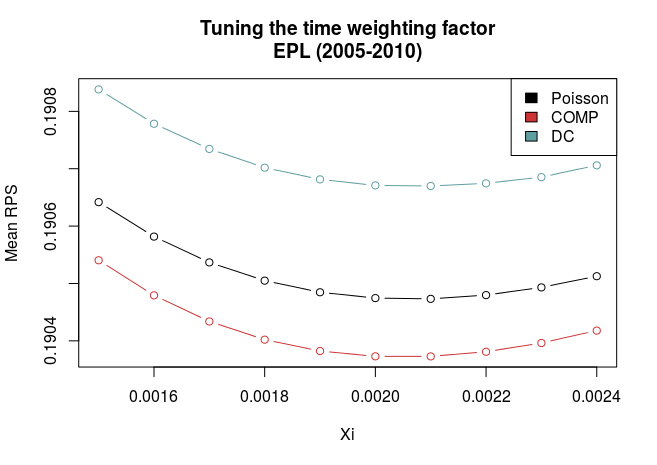The graphs show a couple of things. First, all three models have best predictive ability at the same value of $$\xi$$, and that they compare similarly also for non-optimal values of $$\xi$$. This makes things a bit easier since we don’t have to worry that a different value of $$\xi$$ will alter our evaluations about which model is the best. Second, there is quite some difference between the models for the German and English data. In the English data the COMP model is clearly best, while the DC is the worst. In the German league, the DC is clearly better, and the COMP and Poisson models are pretty much equally good. So I used the optimal values of $$\xi$$ (0.0021 and 0.0015 for Premier League and Bundesliga, respectively) to validate the models in the data from 2010 and onwards. Here is a table of the mean RPS for the three models: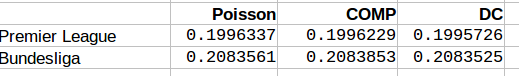We see that for the both English Premier League and German Bundesliga the DC model offers best predictions. The COMP model comes second in Premier League, but has worst performance in the Bundesliga. It is interesting that the DC model performed worst in the tuning period for the Premier League, now was the best one. For the Bundesliga the models compared similarly as in the tuning period. I also looked at how often the DC and COMP models had lower RPS than the Poisson model. The results are in this table: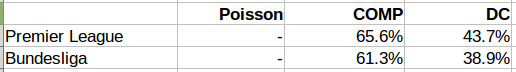The COMP model outperformed the Poisson model in more than 60% of the matches in both leagues, while the DC model did so only about 40% of the time. When looking at the goal scoring probabilities. Here is a table of the sum of the minus log probabilities for the actual scoreline. Here a lower number also indicates better predictions.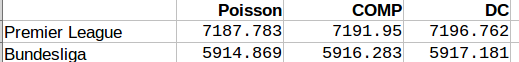Inn both the Premier League and Bundesliga the Poisson model was best, followed by COMP, with the DC model last. We can also take a look at the parameter values for the extra parameters the DC and COMP models has. Remember that the DC models is becomes the Poisson model when rho = 0, while the COMP model is the same as the Poisson model when upsilon = 1, and is underdispersed when upsilon is greater than 1.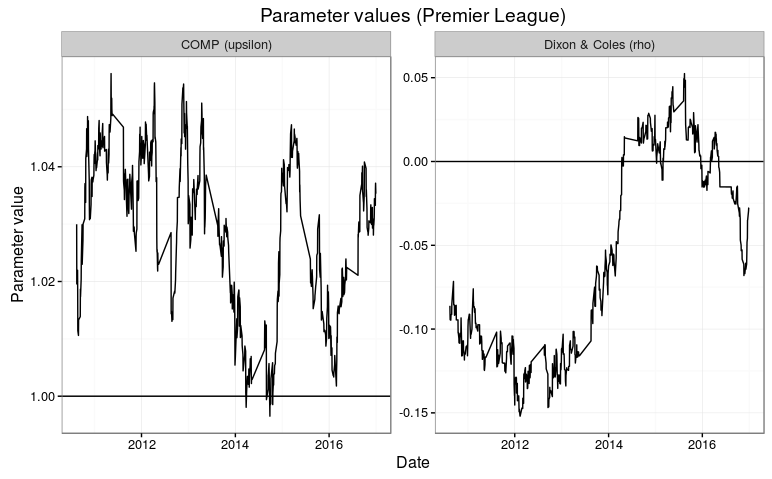The parameter estimates fluctuates a bit. It is intersting to see that the rho parameter in the DC model tend to be below 1, which gives the opposite direction of what Dixon and Coles found in their 1997 paper. In the Premier League, the parmater makes a big jump to above 0 at the end of the 2013-14 season. The parameter appears to be a bit more consistent in the Bundesliga, but also there we see a short period where the parameter is around 0. The dispseriosn parameter upsilon also isn’t all that consistent. It is generally closer to 1 in the Bundesliga than in the Premier League. I think this is consistent with why this model was better in the Premier League than in the Bundesliga. All inn all I think it is hard to conclude which of the three models is the best. The COMP and DC models both adjusts the Poisson model in their own specific ways, and this may explain why the different ways of measuring their predictive abilities are so inconsistent. The DC model seem to be better in the German Bundesliga than in the English Premier League. I don’t think any of the two models are generally better than the ordinary Poisson model, but it could be worthwhile to look more into when the two models are better, and perhaps they could be combined? # The Dixon-Coles approach to time-weighted Poisson regression In the previous blog posts about predicting football results using Poisson regression I have mostly ignored the fact that the data points (ie matches) used to fit the models are gathered (played) at different time points. In the 1997 Dixon and Coles paper where they described the bivariate adjustment for low scores they also discussed using weighted maximum likelihood to better make the parameter estimates reflect the current abilities of the teams, rather than as an average over the whole period you have data from. Dixon and Coles propose to weight the games using a function so that games are down weighted exponentially by how long time it is since they were played. The function to determine the weight for a match played is $$\phi(t) = exp(-\xi t)$$ where t is the time since the match was played, and $$\xi$$ is a positive parameter that determines how much down-weighting should occur. I have implemented this function in R, but I have done a slight modification from the one from the paper. Dixon and Coles uses “half weeks” as their time unit, but they do not describe in more detail what exactly they mean. They probably used Wednesday or Thursday as the day a new half-week starts, but I won’t bother implementing something like that. Instead I am just going to use days as the unit of time. This function takes a vector of the match dates (data type Date) and computes the weights according to the current date and a value of $$\xi$$. The currentDate argument lets you set the date to count from, with all dates after this will be given weight 0. DCweights <- function(dates, currentDate=Sys.Date(), xi=0){ datediffs <- dates - as.Date(currentDate) datediffs <- as.numeric(datediffs *-1) w <- exp(-1*xi*datediffs) w[datediffs <= 0] <- 0 #Future dates should have zero weights return(w) }  We can use this function to plot how the much weight the games in the past is given for different values of $$\xi$$. Here we see that $$\xi = 0$$ gives the same weight to all the matches. I have also set the currentDate as a day in April 2013 to illustrate how future dates are weighted 0.To figure out the optimal value for $$\xi$$ Dixon and Coles emulated a situation where they predicted the match results using only the match data prior to the match in question, and then optimizing for prediction ability. They don’t explain how exactly they did the optimizing, but I am not going to use the optim function to do this. Instead I am going to just try a lot of different values of $$\xi$$, and go for the one that is best. The reason for this is that doing the prediction emulation takes some time, and using an optimizing algorithm will take an unpredictable amount of time. I am not going to use the Dixon-Coles model here. Instead I am going for the independent Poisson model. Again, the reason is that I don’t want to use too much time on this. To measure prediction ability, Dixon & Coles used the predictive log-likelihood (PLL). This is just the logarithm of the probabilities calculated by the model for the outcome that actually occurred, added together for all matches. This means that a greater PLL indicates that the actual outcomes was more probable according to the model, which is what we want. I want to use an additional measure of prediction ability to complement the PLL: The ranked probability score (RPS). This is a measure of prediction error, and takes on values between 0 and 1, with 0 meaning perfect prediction. RPS measure takes into account the entire probability distribution for the three outcomes, not just the probability of the observed outcome. That means a high probability of a draw is considered less of an error that a high probability of away win, if the actual outcome was home win. This measure was popularized in football analytics by Anthony Constantinou in his paper Solving the Problem of Inadequate Scoring Rules for Assessing Probabilistic Football Forecast Models. You can find a link to a draft version of that paper on Constantinou’s website. I used data from the 2005-06 season and onwards, and did predictions from January 2007 and up until the end of 2014. I also skipped the ten first match days at the beginning of each season to avoid problems with lack of data for the promoted teams. I did this for the top leagues in England, Germany, Netherlands and France. Here are optimal values of $$\xi$$ according to the two prediction measurements: PLL RPS England 0.0018 0.0018 Germany 0.0023 0.0023 Netherlands 0.0019 0.0020 France 0.0019 0.0020 The RPS and PLL mostly agree, and where they disagree, it is only by one in the last decimal place. Dixon & Coles found an optimal value of 0.0065 in their data (which were from the 1990s and included data from the top four English leagues and the FA cup), but they used half weeks instead of days as their time unit. Incidentally, if we divide their value by the number of days in a half week (3.5 days) we get 0.00186, about the same I got. The German league has the greatest optimum value, meaning historical data is of less importance when making predictions. An interesting thing to do is to plot the predictive ability against different values of $$\xi$$. Here are the PLL (adjusted to be between 0 and 1, with the optimum at 1) for England and Germany compared, with their optima indicated by the dashed vertical lines:I am not sure I want to interpret this plot too much, but it does seems like predictions for the German league are more robust to values of $$\xi$$ greater than the optimum, as indicated by the slower decline in the graph, than the English league. So here I have presented the values of $$\xi$$ for the independent Poisson regression model, but will these values be the optimal for the Dixon & Coles model? Probably not, but I suspect there will be less variability between the two models than between the same model fitted for different leagues. # The Dixon-Coles model, part 4: A trick to speed up estimation In the previous installments in this series on implementing the Dixon-Coles model I complained a bit about the time it took to estimate the parameters. In the original implementation in part 1 it took about 40 seconds. Now 40 seconds is not much to complain about, there are a whole lot of other models and algorithms that takes much much longer time to fit (for my master’s I had some computations that took several months). Still, I wanted to make a few improvements. The approach I described in part 3 is quite acceptable, I think, especially since it takes less than a second to fit the model. But still, I wanted to make some improvements to my original implementation. There are several reasons for the estimation procedure being slow. I used a general purpose optimizer instead of a tailor-made algorithm, and I didn’t provide the optimizer with a function of the derivative of the model likelihood function, nor the function defining the constraint. This means that the optimizer have to estimate the derivatives by doing a lot of evaluations of the two functions with slight changes in the parameters. The most important speed bump, however, is probably due to how I implemented the constraint that all the average of the attack parameters should equal 1. The alabama package I used relied on a technique called Lagrange multipliers, which is a very general method for constrained optimization. Instead of relying on general constrained optimization procedures, there is a trick commonly used in linear models with sum-to-zero constrained categorical parameters that we also can use here. There has been some discussion and confusion in the comments about how categorical variables are coded and how R presents the results of the glm function. A thorough discussion of this is best left for another time, but let me explain how the sum-to-zero constraint is implemented in linear models. We will fit the model with this constraint and then make some adjustments later on to get the correct average-is-one constraint. The sum-to-zero constraint basically says that the sum of all the parameters for a categorical variable must equal to zero: $$\sum_{i=1} \theta_i = 0$$ If we for example have three levels, we can write out the equation like this: $$\theta_1 + \theta_2 + \theta_3 = 0$$ If we subtract $$\theta_3$$ and multiply both sides of the equation by minus 1 we get $$– \theta_1 – \theta_2 = \theta_3$$ Notice how we can write one of the parameters as a simple linear function of the other parameters. We can use this result to construct the design matrix for the categorical variable, incorporating the sum-to-zero constraint (exactly which parameter or level we chose to be a function of the others doesn’t matter, the end results does not differ). Suppose we have the following observations of a three-level categorical variable: $$\begin{bmatrix} A & A & B & B & C & C \end{bmatrix}^T$$ We can then construct the following design matrix: $$\begin{bmatrix} 1 & 0 \\ 1 & 0 \\ 0 & 1 \\ 0 & 1 \\ -1 & -1 & \\ -1 & -1 & \end{bmatrix}$$ Notice that we only need two columns (i.e. two variables) to encode the three levels. Since the last parameter is a function of the two other it is redundant. Also notice how the observations in the two last rows, corresponding to the $$C$$ observations, will influence the estimation of all the other parameters for this variable. When the two parameters are estimated, the last parameter can be obtained using the result from above relating the last parameter to all the other. In the Dixon-Coles paper they used the constraint that the average of the attack parameters should be 1. This is not quite the same as the sum-to-zero constraint, but for prediction, it does not matter exactly which constraint we use. Anyway, I will explain later how we can fix this. To use this trick in the Dixon-Coles implementation we need to make the following changes to our code from part 1. Obviously the first thing we need to change is how the design matrices in the DCmodelData function is computed. We need four matrices now, since the number of parameters estimated directly is different for the attack and defense parameters. Notice how I chose the last of team that appear last in the team.names vector. The teams get sorted alphabetically, so for the 2011-12 Premier League data this is is Wolves. DCmodelData <- function(df){ team.names <- unique(c(levels(df$HomeTeam), levels(df$AwayTeam))) # attack, with sum-to-zero constraint ## home hm.a <- model.matrix(~ HomeTeam - 1, data=df) hm.a[df$HomeTeam == team.names[length(team.names)], ] <- -1
hm.a <- hm.a[,1:(length(team.names)-1)]

# away
am.a <- model.matrix(~ AwayTeam -1, data=df)
am.a[df$AwayTeam == team.names[length(team.names)], ] <- -1 am.a <- am.a[,1:(length(team.names)-1)] # defence, same as before hm.d <- model.matrix(~ HomeTeam - 1, data=df) am.d <- model.matrix(~ AwayTeam -1, data=df) return(list(homeTeamDMa=hm.a, homeTeamDMd=hm.d, awayTeamDMa=am.a, awayTeamDMd=am.d, homeGoals=df$FTHG, awayGoals=df$FTAG, teams=team.names)) }  Some changes to the DCoptimFn function is also needed, so it properly handles the changes we made to the design matrices. # I don't bother showing the rest of the function nteams <- length(DCm$teams)
attack.p <- matrix(params[3:(nteams+1)], ncol=1) #one column less
defence.p <- matrix(params[(nteams+2):length(params)], ncol=1)

# need to multiply with the correct matrices
lambda <- exp(DCm$homeTeamDMa %*% attack.p + DCm$awayTeamDMd %*% defence.p + home.p)
mu <- exp(DCm$awayTeamDMa %*% attack.p + DCm$homeTeamDMd %*% defence.p)


We also need to make a the appropriate adjustments to the vectors with the initial parameter values, so that they have the correct lengths.

dcm <- DCmodelData(data)
nteams <- length(dcm$teams) #initial parameter estimates attack.params <- rep(.1, times=nteams-1) # one less parameter defence.params <- rep(-0.8, times=nteams) home.param <- 0.06 rho.init <- 0.03 par.inits <- c(home.param, rho.init, attack.params, defence.params) #informative names #skip the last team names(par.inits) <- c('HOME', 'RHO', paste('Attack', dcm$teams[1:(nteams-1)], sep='.'),
paste('Defence', dcm$teams, sep='.'))  With these changes we can simply use the built-in optim function in R. There is no need for the DCattackConstr function anymore, or a third party package, since we built the constraint right into the design matrices. res <- optim(par=par.inits, fn=DCoptimFn, DCm=dcm, method='BFGS')  This takes about 6-7 seconds on my laptop, a decent improvement to the 40 seconds it took before. If you take a look at the resulting parameter estimates in res$par you will see that the attack parameter for Wolves is missing. As I explained earlier, this parameter is easy to find. It is also easy to correct all the parameter estimates so that they become the same as if we had a mean-is-one constraint on the attack parameters. This is done by increasing the attack parameters by one, and decreasing the defense parameters by one. The reason it is that simple is that the sum-to-zero constraint is equivalent with a mean-is-zero constraint.

parameters <- res$par #compute Wolves attack parameter missing.attack <- sum(parameters[3:(nteams+1)]) * -1 #put it in the parameters vector parameters <- c(parameters[1:(nteams+1)], missing.attack, parameters[(nteams+2):length(parameters)]) names(parameters)[nteams+2] <- paste('Attack.', dcm$teams[nteams], sep='')

#increase attack by one
parameters[3:(nteams+2)] <- parameters[3:(nteams+2)] + 1

#decrease defence by one
parameters[(nteams+3):length(parameters)] <- parameters[(nteams+3):length(parameters)] - 1


# The Dixon-Coles model for predicting football matches in R (part 3)

About a moth ago Martin Eastwood of the pena.lt/y blog put up some slides from a talk he gave about predicting football results in R. He presented in detail the independent Poisson regression model, and how to implement it. He also briefly mentioned and showed the bivariate adjustments in the Dixon-Coles model. I was curious about how he had implemented it since I had just finished my own implementation. In the comments he said that he used a two-stage approach, first estimating the attack and defense parameters using the independent Poisson model, and then estimating the rho parameter by it self.

This method may be less accurate than fitting the complete model, but it will probably be more accurate than the independent Poisson model. It is without a doubt faster and easier to implement.

We start with loading the data, and then making a new data.frame that contains two rows per match, as described in my post about the independent Poisson model.

dta <- read.csv('FAPL1112.csv')

# Data formated for the independent model
# Store in new variable, we need the data in original format later
dta.indep <- data.frame(Team=as.factor(c(as.character(dta$HomeTeam), as.character(dta$AwayTeam))),
Opponent=as.factor(c(as.character(dta$AwayTeam), as.character(dta$HomeTeam))),
Goals=c(dta$FTHG, dta$FTAG),
Home=c(rep(1, dim(dta)), rep(0, dim(dta))))



Now fit the model:

m <- glm(Goals ~ Home + Team + Opponent, data=dta.indep, family=poisson())


Since we now have estimated the attack, defense and home parameters we can use the built-in functions in R to calculate the expected home and away scores (lambda and mu).

To calculate lambda and mu, we use the fitted function. I organized the data so that all the rows with the goals scored by the home team comes before all the rows with the goals by the away teams. Whats more, the match in the first row in the home team part corresponds to the match in the first row in the away team part, so it is easy to get the corresponding expectations correct.

expected <- fitted(m)
home.expected <- expected[1:nrow(dta)]
away.expected <- expected[(nrow(dta)+1):(nrow(dta)*2)]


To estimate the rho parameter we can use the tau and DClogLik function we defined in part 1. We just wrap it inside a function we pass to the built in optimizer in R:

DCoptimRhoFn <- function(par){
rho <- par
DClogLik(dta$FTHG, dta$FTAG, home.expected, away.expected, rho)
}

res <- optim(par=c(0.1), fn=DCoptimRhoFn, control=list(fnscale=-1), method='BFGS')


The optimization finishes in an instant. As before we get the parameter values by looking at res$par. The estimated rho parameter is -0.126, which is reassuringly not that different from the -0.134 we got from the full model. This is is also about the same values Justin Worrall gets at his sportshacker.net blog. To make predictions we can reuse most of the code from part 2. The only substantial difference is how we calculate the expected goals, which is a bit simpler this time: # Expected goals home lambda <- predict(m, data.frame(Home=1, Team='Bolton', Opponent='Blackburn'), type='response') # Expected goals away mu <- predict(m, data.frame(Home=0, Team='Blackburn', Opponent='Bolton'), type='response')  This two-stage approach is much faster and simpler. We don’t have to manually create the design matrices and use matrix algebra to calculate the expected scores. We also don’t have to write as much code to keep track of all the parameters. I haven’t really compared all the different models against each other, so I can’t say which one makes the best predictions, but my guess is that this two-stage approach gives results similar to the fully specified Dixon-Coles model. # The Dixon-Coles model for predicting football matches in R (part 2) Part 1 ended with running the optimizer function to estimate the parameters in the model: library(alabama) res <- auglag(par=par.inits, fn=DCoptimFn, heq=DCattackConstr, DCm=dcm) # Take a look at the parameters res$par


In part 1 I fitted the model to data from the 2011-12 Premier League season. Now it’s time to use the model to make a prediction. As an example I will predict the result of Bolton playing at home against Blackburn.

The first thing we need to do is to calculate the mu and lambda parameters, which is (approximately anyway) the expected number of goals scored by the home and away team. To do this wee need to extract the correct parameters from the res$par vector. Recall that I in the last post gave the parameters informative names that consists of the team name prefixed by either Attack or Defence. Also notice that I have to multiply the team parameters and then exponentiate the result to get the correct answer. Update: For some reason I got the idea that the team parameters should be multiplied together, instead of added together, but I have now fixed the code and the results. # Expected goals home lambda <- exp(res$par['HOME'] + res$par['Attack.Bolton'] + res$par['Defence.Blackburn'])

# Expected goals away
mu <- exp(res$par['Attack.Blackburn'] + res$par['Defence.Bolton'])


We get that Bolton is expected to score 2.07 goals and Blackburn is expected to score 1.59 goals.

Since the model assumes dependencies between the number of goals scored by the two teams, it is insufficient to just plug the lambda and mu parameters into R’s built-in Poisson function to get the probabilities for the number of goals scored by the two teams. We also need to incorporate the adjustment for the low-scoring results as well. One strategy to do this is to first create a matrix based on the simple independent Poisson distributions:

maxgoal <- 6 # will be useful later
probability_matrix <- dpois(0:maxgoal, lambda) %*% t(dpois(0:maxgoal, mu))


The number of home goals follows the vertical axis and the away goals follow the horizontal.

Now we can use the estimated dependency parameter rho to create a 2-by-2 matrix with scaling factors, that is then element-wise multiplied with the top left elements of the matrix calculated above:

Update: Thanks to Mike who pointed out a mistake in this code.

scaling_matrix <- matrix(tau(c(0,1,0,1), c(0,0,1,1), lambda, mu, res$par['RHO']), nrow=2) probability_matrix[1:2, 1:2] <- probability_matrix[1:2, 1:2] * scaling_matrix  With this matrix it is easy to calculate the probabilities for the three match outcomes: HomeWinProbability <- sum(probability_matrix[lower.tri(probability_matrix)]) DrawProbability <- sum(diag(probability_matrix)) AwayWinProbability <- sum(probability_matrix[upper.tri(probability_matrix)])  This gives a probability of 0.49 for home win, 0.21 for draw and 0.29 for away win. Calculating the probabilities for the different goal differences is a bit trickier. The probabilities for each goal difference can be found by adding up the numbers on the diagonals, with the sum of the main diagonal being the probability of a draw. awayG <- numeric(maxgoal) for (gg in 2:maxgoal){ awayG[gg-1] <- sum(diag(probability_matrix[,gg:(maxgoal+1)])) } awayG[maxgoal] <- probability_matrix[1,(maxgoal+1)] homeG <- numeric(maxgoal) for (gg in 2:maxgoal){ homeG[gg-1] <- sum(diag(probability_matrix[gg:(maxgoal+1),])) } homeG[maxgoal] <- probability_matrix[(maxgoal+1),1] goaldiffs <- c(rev(awayG), sum(diag(probability_matrix)), homeG) names(goaldiffs) <- -maxgoal:maxgoal  It is always nice to plot the probability distribution: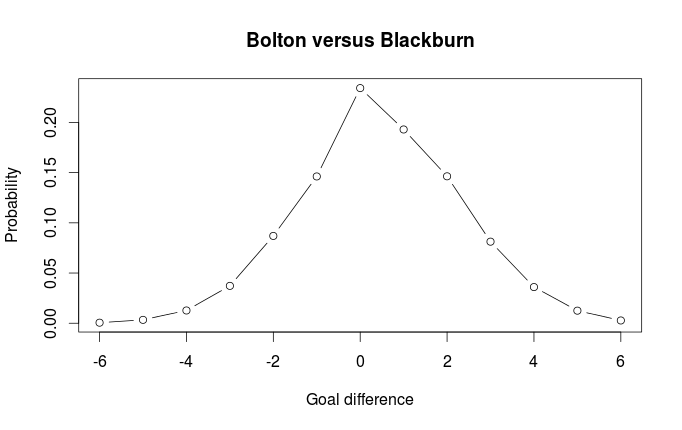We can also see compare this distribution with the distribution without the Dixon-Coles adjustment (i.e. the goals scored by the two teams are independent):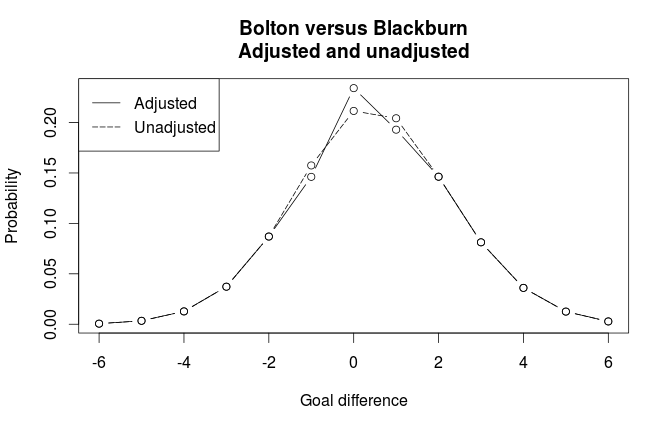As expected, we see that the adjustment gives higher probability for draw, and lower probabilities for goal differences of one goal. # The Dixon-Coles model for predicting football matches in R (part 1) Please have a look at the improved code for this model that I have posted here. When it comes to Poisson regression models for football results, the 1997 paper Modelling Association Football Scores and Inefficiencies in the Football Betting Market (pdf) by Dixon and Coles is often mentioned. In this paper the authors describe an improvement of the independent goals model. The improvement consists of modeling a dependence between the probabilities for the number of goals less than 2 for both teams. They also improve the model by incorporating a time perspective, so that matches played a long time a go does not have as much influence on the parameter estimates. The model by Dixon and Coles is not as easy to fit as the independent Poisson model I have described earlier. There is no built-in function in R that can estimate it’s parameters, and the authors provide little details about how to implement it. Mostly as an exercise, I have implemented the model in R, but without the time down-weighting scheme. The estimating procedure uses a technique called maximum likelihood. This is perhaps the most commonly used method for estimating parameters in statistical models. The way it works is that you specify a way to calculate the likelihood of your data for a given set of parameters, and then you need to find the set of parameters that gives the highest possible likelihood of your data. The independent Poisson model is also fitted using a maximum likelihood method. The difference here is that the likelihood used by Dixon and Coles is non-standard. The model is pretty much similar to other regression models I have discussed. Each team has an attack and a defense parameter, and from a function of these the expected number of goals for each team in a match is calculated. For the rest of this post I am going to assume you have read the paper. There is a link to it in the first paragraph. The most obvious thing we have to do is to implement the function referred to by the greek letter Tau. This is the function that, dependent on the Rho parameter, computes the degree in which the probabilities for the low scoring goals changes. tau <- Vectorize(function(xx, yy, lambda, mu, rho){ if (xx == 0 & yy == 0){return(1 - (lambda*mu*rho)) } else if (xx == 0 & yy == 1){return(1 + (lambda*rho)) } else if (xx == 1 & yy == 0){return(1 + (mu*rho)) } else if (xx == 1 & yy == 1){return(1 - rho) } else {return(1)} })  We can now make a function for the likelihood of the data. A common trick when implementing likelihood functions is to use the log-likelihood instead. The reason is that when the probabilities for each data point for a given set of parameters are multiplied together, they will be too small for the computer to handle. When the probabilities are log-transformed you can instead just add them together. What this function does is that it takes the vectors of mu (expected home goals) and lambda (expected away goals), Rho, and the vectors of observed home and away goals, and computes the log-likelihood for all the data. DClogLik <- function(y1, y2, lambda, mu, rho=0){ #rho=0, independence #y1: home goals #y2: away goals sum(log(tau(y1, y2, lambda, mu, rho)) + log(dpois(y1, lambda)) + log(dpois(y2, mu))) }  The team specific attack and defense parameters are not included in the log-likelihood function. Neither is the code that calculates the expected number of goals for each team in a match (lambda and mu). Before we can calculate these for each match, we need to do some data wrangling. Here is a function that takes a data.frame formated like the data from football-data.co.uk, and returns a list with design matrices and vectors with the match results. DCmodelData <- function(df){ hm <- model.matrix(~ HomeTeam - 1, data=df, contrasts.arg=list(HomeTeam='contr.treatment')) am <- model.matrix(~ AwayTeam -1, data=df) team.names <- unique(c(levels(df$HomeTeam), levels(df$AwayTeam))) return(list( homeTeamDM=hm, awayTeamDM=am, homeGoals=df$FTHG,
awayGoals=df$FTAG, teams=team.names )) }  Now we create a function that calculates the log-likelihod from a set of parameters and the data we have. First it calculates the values for lambda and mu for each match, then it passes these and the number of goals scored in each match to the log-likelihood function. This function needs to be written in such a way that it can be used by another function that will find the parameters that maximizes the log-likelihood. First, all the parameters needs to be given to a single argument in the form of a vector (the params argument). Also, the log-likelihood is multiplied by -1, since the optimization function we are going to use only minimizes, but we want to maximize. DCoptimFn <- function(params, DCm){ home.p <- params rho.p <- params nteams <- length(DCm$teams)
attack.p <- matrix(params[3:(nteams+2)], ncol=1)
defence.p <- matrix(params[(nteams+3):length(params)], ncol=1)

lambda <- exp(DCm$homeTeamDM %*% attack.p + DCm$awayTeamDM %*% defence.p + home.p)
mu <- exp(DCm$awayTeamDM %*% attack.p + DCm$homeTeamDM %*% defence.p)

return(
DClogLik(y1=DCm$homeGoals, y2=DCm$awayGoals, lambda, mu, rho.p) * -1
)
}


One more thing we need before we start optimizing is a function that helps the optimizer handle the constraint that all the attack parameters must sum to 1. If this constraint isn’t given, it will be impossible to find a unique set of parameters that maximizes the likelihood.

DCattackConstr <- function(params, DCm, ...){
nteams <- length(DCm$teams) attack.p <- matrix(params[3:(nteams+2)], ncol=1) return((sum(attack.p) / nteams) - 1) }  Now we are finally ready to find the parameters that maximizes the likelihood based on our data. First, load the data (in this case data from the 2011-12 premier league), and properly handle it with our DCmodelData function: dta <- read.csv('FAPL1112.csv') dcm <- DCmodelData(dta)  Now we need to give a set of initial estimates of our parameters. It is not so important what specific values these are, but should preferably be in the same order of magnitude as what we think the estimated parameters should be. I set all attack parameters to 0.1 and all defense parameters to -0.8. #initial parameter estimates attack.params <- rep(.01, times=nlevels(dta$HomeTeam))
defence.params <- rep(-0.08, times=nlevels(dta$HomeTeam)) home.param <- 0.06 rho.init <- 0.03 par.inits <- c(home.param, rho.init, attack.params, defence.params) #it is also usefull to give the parameters some informative names names(par.inits) <- c('HOME', 'RHO', paste('Attack', dcm$teams, sep='.'), paste('Defence', dcm$teams, sep='.'))  To optimize with equality constraints (all attack parameters must sum to 1) we can use the auglag function in the alabama package. This takes about 40 seconds to run on my laptop, much longer than the independent Poisson model fitted with the built in glm function. This is because the auglag function uses some general purpose algorithms that can work with a whole range of home-made functions, while the glm function is implemented with a specific set of models in mind. library(alabama) res <- auglag(par=par.inits, fn=DCoptimFn, heq=DCattackConstr, DCm=dcm)  Voilà! Now the parameters can be found by the command res$par. In a follow-up post I will show how we can use the model to make prediction of match outcomes.

Team Attack Defence
Arsenal 1.37 -0.91
Aston Villa 0.69 -0.85
Blackburn 0.94 -0.47
Bolton 0.92 -0.48
Chelsea 1.23 -0.97
Everton 0.94 -1.15
Fulham 0.93 -0.89
Liverpool 0.89 -1.13
Man City 1.56 -1.43
Man United 1.52 -1.31
Newcastle 1.10 -0.88
Norwich 1.02 -0.62
QPR 0.82 -0.65
Stoke 0.64 -0.87
Sunderland 0.86 -0.99
Swansea 0.85 -0.89
Tottenham 1.24 -1.09
West Brom 0.86 -0.88
Wigan 0.81 -0.71
Wolves 0.79 -0.42
Home 0.27
Rho -0.13